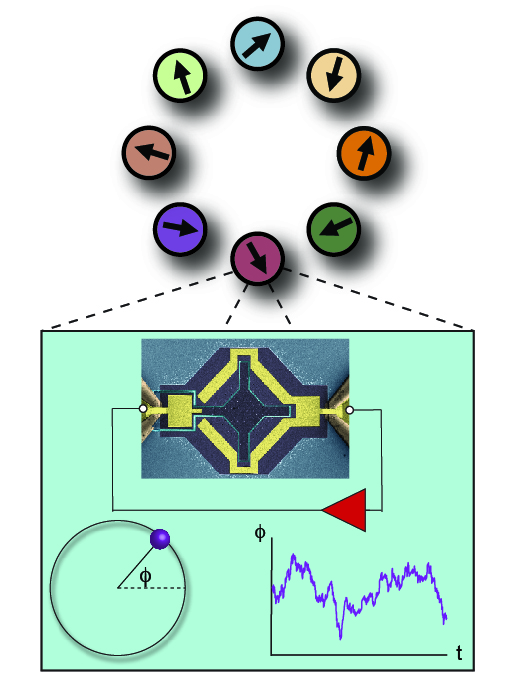## Home / Research

### Movies for "Exotic states in a simple network of nanoelectromechanical oscillators"

The following videos are integral parts to my upcoming manuscript "Exotic states in a simple network of nanoelectromechanical oscillators". Each one shows real-time data from an 8-ring of nanomechanical oscillators. The arrows in the videos reflect data of oscillator values. the length is the the amplitude of osclliation, and the angle with respect to horizontal is the phase of the oscillation.

In this video, we show an example of synchronization. In the beginning, no apparent phase order is seen at any point in time and the oscillators are not synchronized. This is because the oscillators are uncoupled. However, in this system we have control over coupling. As we turn on the coupling we observe a stark transition to an antiphase synchronized state.
In this video, we show an example of a synchronized splay state. This is the k=7 state - in other words the phase differences between nearest-neighbors is 7π/4. Splay states are also known as 'rotating wave' states because the phase travels around the ring. Here, the phase travels in a counter-clockwise direction. This state has a 8-fold twisted rotational symmetry. This twist is given by the k number, and represents the amount of phase rotation that must accompany the spatial ring rotation. Since phase tracks time, by running forward time, we can run forward phase. As an example, a phase rotation by 7π/4 is accompanined by a spatial rotation of the ring by pi/4. To see this we run forward time by 7/8 of a cycle, after which, we now see the ring has indeed rotated by one oscillator, or pi/4.
In this video, we show pattern formation over oscillator phases. The pattern we discuss here arises out of a uniform synchronized state with identical oscillator phases, i.e. the in-phase state. The in-phase state is only stable when network coupling is large. If we suddenly shift this coupling down beyond where the state is stable we quench the system. After the quench, the system displays a a spread in the phase, which is not random. The phase winds up and down with a spatial period of 8 oscillators.
In this video, we show oscillator decoupling, here seen for the first time in experiment. We see two clusters of oscillators, one that precesses slowly over long times, and one that does not precess at all. Note the oscillators only have physical coupling between nearest neighbors. However, emergent second order effects show up as physical coupling increases, giving next-nearest neighbor interactions.
In this video, we examine a weak chimera in our system. Weak chimeras are synchronized states with a cluster of oscillators not synchronized to other oscillators. We see two independent clusters in this state and they rotate in opposite directions, so they are clearly not synchronized. These clusters contain in-phase and antiphase pairs, located across the ring. This type of state appears in systems of phase oscillators with biharmonic coupling. This interaction can emerge in phase oscillators as the physical coupling between real oscillators increases. So how do opposite pairs synchronize with only nearest neighbor coupling? The antiphase pairs interact with the in-phase pairs. Thus, an effective interaction can be mediated by the unsynchronized pairs.
In this video, we show another weak chimera. This state reveals a dynamical symmetry in the system not related to the octogonal symmetry of the spatial layout, or to the continuous temporal symmetry associated with the oscillator phase. We see instantaneous phase correlations in pairs of oscillators, and the symmetry given by those correlations gives a subgroup of the original symmetry group. However, there is additional 'delayed' correlation between oscillators across a reflection line. This is a symmetry which arises from the particular dynamics of this system, which is more complicated than a simple ring network of phase oscillators.